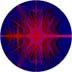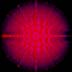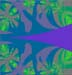#### More Colorings of the Unit Disk Based on an Almost Colinear Condition

Given epsilon > 0, define the color of the complex number z to be the smallest positive integer n such that |(z - 1).im / (z - 1).re - (zn - 1).im / (zn - 1).re| < epsilon. That is, the slope of the line through z and 1 differs from the slope of the line through zn and 1 by less than epsilon.© Harriet Fell 1994 epsilon = 0.01 maximum power of z = 10© Harriet Fell 1994 epsilon = 0.1 maximum power of z = 16© Harriet Fell 1994 epsilon = 0.01 maximum power of z = 16© Harriet Fell 1994 epsilon = 0.001 maximum power of z = 16© Harriet Fell 1994 epsilon = 0.3 maximum power of z = 32© Harriet Fell 1994 epsilon = 0.1 maximum power of z = 32© Harriet Fell 1994 epsilon = 0.01 maximum power of z = 32

Harriet J. Fell
College of Computer Science
Northeastern University, Boston, MA 02115
Phone: (617) 373-2198
fell@ccs.neu.edu

The URL for this document is: http://www.ccs.neu.edu/home/fell/colinearColorings.html